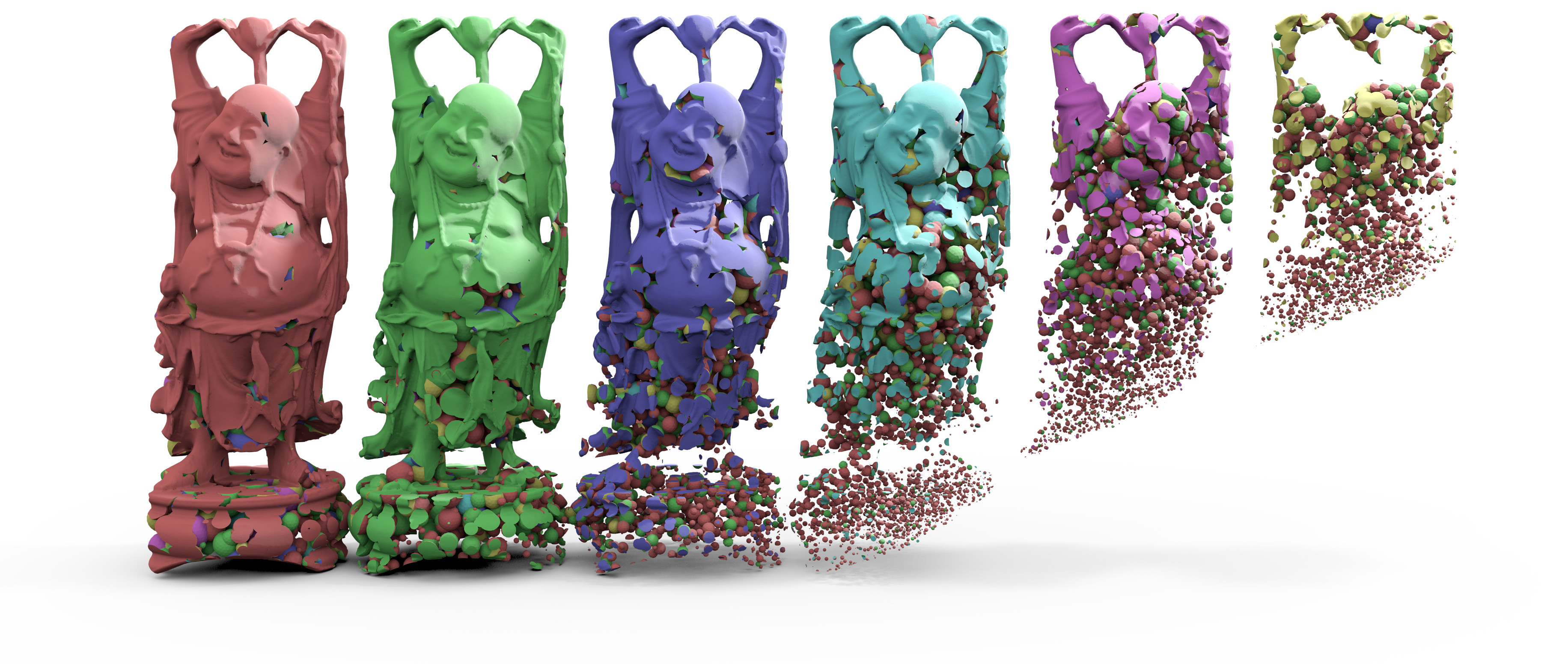EN FR
EN FR

## Section: New Results

### QuickCSG: Arbitrary and Faster Boolean Combinations of N Solids

While studied over several decades, the computation of boolean operations on polyhedra is almost always addressed by focusing on the case of two polyhedra. For multiple input polyhedra and an arbitrary boolean operation to be applied, the operation is decomposed over a binary CSG tree, each node being processed separately in quasilinear time. For large trees, this is both error prone due to intermediate geometry and error accumulation, and inefficient because each node yields a specific overhead. We introduce a fundamentally new approach to polyhedral CSG evaluation, addressing the general N-polyhedron case. We propose a new vertex-centric view of the problem, which both simplifies the algorithm computing resulting geometric contributions, and vastly facilitates its spatial decomposition. We then embed the entire problem in a single KD-tree, specifically geared toward the final result by early pruning of any region of space not contributing to the final surface. This not only improves the robustness of the approach, it also gives it a fundamental speed advantage, with an output complexity depending on the output mesh size instead of the input size as with usual approaches. Complemented with a task-stealing parallelization, the algorithm achieves breakthrough performance, one to two orders of magnitude speedups with respect to state-of-the-art CPU algorithms, on boolean operations over two to several dozen polyhedra. The algorithm is also shown to outperform recent GPU implementations and approximate discretizations, while producing a topologically exact output without redundant facets. This algorithm was published as Inria research report  .

Figure 3. Intersection of 6 Buddhas with the union of 100,000 spheres (total 24 million triangles). Computed in 8 seconds on a desktop machine